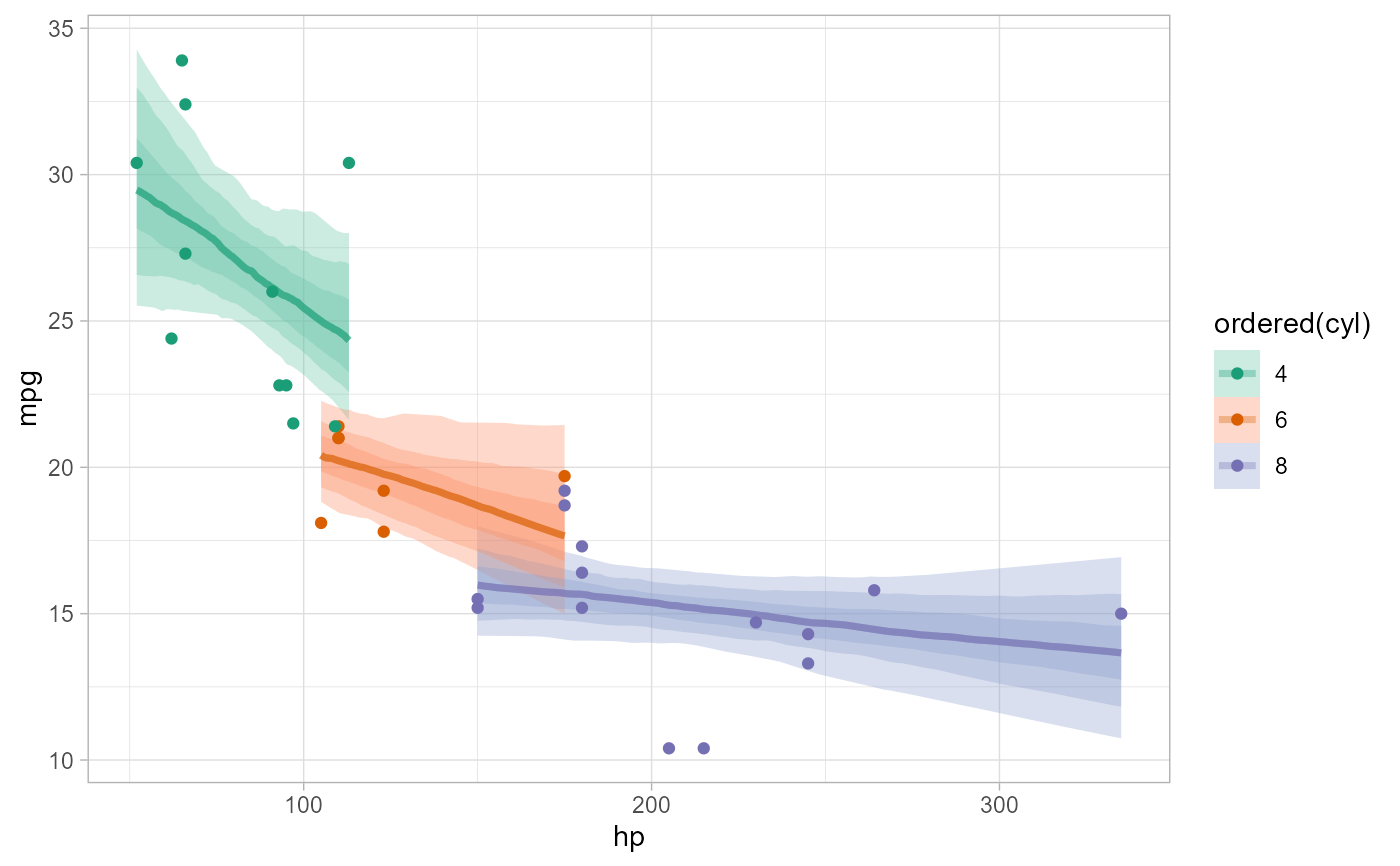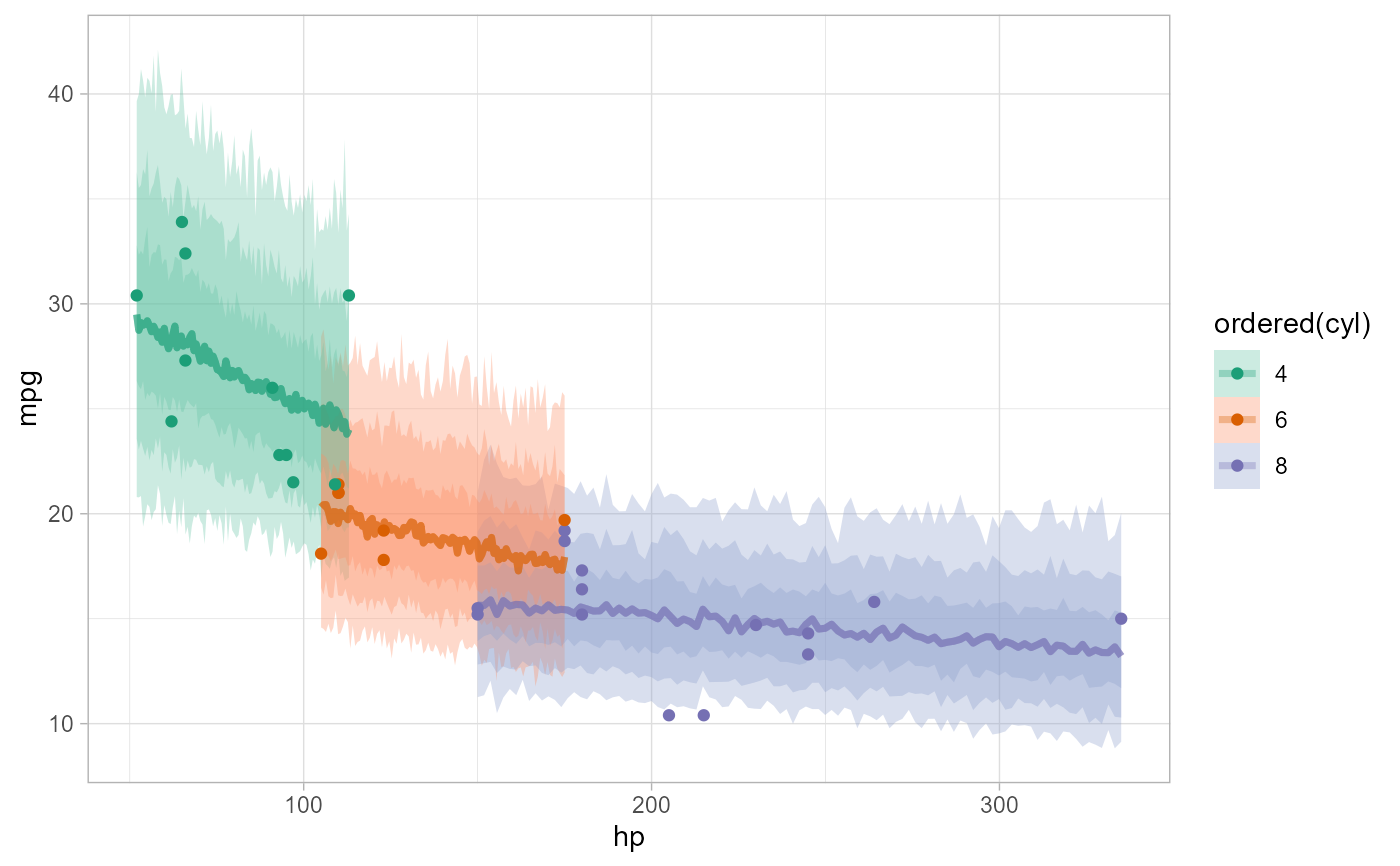Given a data frame and a model, adds rvars of draws from the linear/link-level predictor, the expectation of the posterior predictive, or the posterior predictive to the data frame.

add_epred_rvars(
newdata,
object,
...,
value = ".epred",
ndraws = NULL,
seed = NULL,
re_formula = NULL,
dpar = NULL,
columns_to = NULL
)

epred_rvars(
object,
newdata,
...,
value = ".epred",
ndraws = NULL,
seed = NULL,
re_formula = NULL,
dpar = NULL,
columns_to = NULL
)

# S3 method for default
epred_rvars(
object,
newdata,
...,
value = ".epred",
seed = NULL,
dpar = NULL,
columns_to = NULL
)

# S3 method for stanreg
epred_rvars(
object,
newdata,
...,
value = ".epred",
ndraws = NULL,
seed = NULL,
re_formula = NULL,
dpar = NULL,
columns_to = NULL
)

# S3 method for brmsfit
epred_rvars(
object,
newdata,
...,
value = ".epred",
ndraws = NULL,
seed = NULL,
re_formula = NULL,
dpar = NULL,
columns_to = NULL
)

newdata,
object,
...,
value = ".linpred",
ndraws = NULL,
seed = NULL,
re_formula = NULL,
dpar = NULL,
columns_to = NULL
)

linpred_rvars(
object,
newdata,
...,
value = ".linpred",
ndraws = NULL,
seed = NULL,
re_formula = NULL,
dpar = NULL,
columns_to = NULL
)

# S3 method for default
linpred_rvars(
object,
newdata,
...,
value = ".linpred",
seed = NULL,
dpar = NULL,
columns_to = NULL
)

# S3 method for stanreg
linpred_rvars(
object,
newdata,
...,
value = ".linpred",
ndraws = NULL,
seed = NULL,
re_formula = NULL,
dpar = NULL,
columns_to = NULL
)

# S3 method for brmsfit
linpred_rvars(
object,
newdata,
...,
value = ".linpred",
ndraws = NULL,
seed = NULL,
re_formula = NULL,
dpar = NULL,
columns_to = NULL
)

newdata,
object,
...,
value = ".prediction",
ndraws = NULL,
seed = NULL,
re_formula = NULL,
columns_to = NULL
)

predicted_rvars(
object,
newdata,
...,
value = ".prediction",
ndraws = NULL,
seed = NULL,
re_formula = NULL,
columns_to = NULL
)

# S3 method for default
predicted_rvars(
object,
newdata,
...,
value = ".prediction",
seed = NULL,
columns_to = NULL
)

# S3 method for stanreg
predicted_rvars(
object,
newdata,
...,
value = ".prediction",
ndraws = NULL,
seed = NULL,
re_formula = NULL,
columns_to = NULL
)

# S3 method for brmsfit
predicted_rvars(
object,
newdata,
...,
value = ".prediction",
ndraws = NULL,
seed = NULL,
re_formula = NULL,
columns_to = NULL
)

## Arguments

newdata

Data frame to generate predictions from.

object

A supported Bayesian model fit that can provide fits and predictions. Supported models are listed in the second section of tidybayes-models: Models Supporting Prediction. While other functions in this package (like spread_rvars()) support a wider range of models, to work with add_epred_rvars(), add_predicted_rvars(), etc. a model must provide an interface for generating predictions, thus more generic Bayesian modeling interfaces like runjags and rstan are not directly supported for these functions (only wrappers around those languages that provide predictions, like rstanarm and brm, are supported here).

...

Additional arguments passed to the underlying prediction method for the type of model given.

value

The name of the output column:

• for [add_]epred_rvars(), defaults to ".epred".

• for [add_]predicted_rvars(), defaults to ".prediction".

• for [add_]linpred_rvars(), defaults to ".linpred".

ndraws

The number of draws to return, or NULL to return all draws.

seed

A seed to use when subsampling draws (i.e. when ndraws is not NULL).

re_formula

formula containing group-level effects to be considered in the prediction. If NULL (default), include all group-level effects; if NA, include no group-level effects. Some model types (such as brms::brmsfit and rstanarm::stanreg-objects) allow marginalizing over grouping factors by specifying new levels of a factor in newdata. In the case of brms::brm(), you must also pass allow_new_levels = TRUE here to include new levels (see brms::posterior_predict()).

dpar

For add_epred_rvars() and add_linpred_rvars(): Should distributional regression parameters be included in the output? Valid only for models that support distributional regression parameters, such as submodels for variance parameters (as in brms::brm()). If TRUE, distributional regression parameters are included in the output as additional columns named after each parameter (alternative names can be provided using a list or named vector, e.g. c(sigma.hat = "sigma") would output the "sigma" parameter from a model as a column named "sigma.hat"). If NULL or FALSE (the default), distributional regression parameters are not included.

columns_to

For some models, such as ordinal, multinomial, and multivariate models (notably, brms::brm() models but not rstanarm::stan_polr() models), the column of predictions in the resulting data frame may include nested columns. For example, for ordinal/multinomial models, these columns correspond to different categories of the response variable. It may be more convenient to turn these nested columns into rows in the output; if this is desired, set columns_to to a string representing the name of a column you would like the column names to be placed in. In this case, a .row column will also be added to the result indicating which rows of the output correspond to the same row in newdata. See vignette("tidy-posterior") for examples of dealing with output ordinal models.

## Value

A data frame (actually, a tibble) equal to the input newdata with additional columns added containing rvars representing the requested predictions or fits.

## Details

add_epred_rvars() adds rvars containing draws from the expectation of the posterior predictive distribution to the data. It corresponds to rstanarm::posterior_epred() or brms::posterior_epred().

add_predicted_rvars() adds rvars containing draws from the posterior predictive distribution to the data. It corresponds to rstanarm::posterior_predict() or brms::posterior_predict().

add_linpred_rvars() adds rvars containing draws from the (possibly transformed) posterior linear predictors (or "link-level" predictors) to the data. It corresponds to rstanarm::posterior_linpred() or brms::posterior_linpred().

The corresponding functions without add_ as a prefix are alternate spellings with the opposite order of the first two arguments: e.g. add_predicted_rvars() and predicted_rvars(). This facilitates use in data processing pipelines that start either with a data frame or a model.

Given equal choice between the two, the spellings prefixed with add_ are preferred.

add_predicted_draws() for the analogous functions that use a long-data-frame-of-draws format instead of a data-frame-of-rvars format. See spread_rvars() for manipulating posteriors directly.

Matthew Kay

## Examples

# \donttest{

library(ggplot2)
library(dplyr)
library(posterior)
#> This is posterior version 1.1.0.9000
#>
#> Attaching package: 'posterior'
#> The following object is masked from 'package:brms':
#>
#>     rhat
#> The following objects are masked from 'package:stats':
#>
library(brms)
library(modelr)

theme_set(theme_light())

m_mpg = brm(mpg ~ hp * cyl, data = mtcars, family = lognormal(),
# 1 chain / few iterations just so example runs quickly
# do not use in practice
chains = 1, iter = 500)
#> Compiling Stan program...
#> Start sampling
#>
#> SAMPLING FOR MODEL 'b46882bb7b271f19aeb621c75c1dd384' NOW (CHAIN 1).
#> Chain 1:
#> Chain 1: Gradient evaluation took 0.001 seconds
#> Chain 1: 1000 transitions using 10 leapfrog steps per transition would take 10 seconds.
#> Chain 1:
#> Chain 1:
#> Chain 1: Iteration:   1 / 500 [  0%]  (Warmup)
#> Chain 1: Iteration:  50 / 500 [ 10%]  (Warmup)
#> Chain 1: Iteration: 100 / 500 [ 20%]  (Warmup)
#> Chain 1: Iteration: 150 / 500 [ 30%]  (Warmup)
#> Chain 1: Iteration: 200 / 500 [ 40%]  (Warmup)
#> Chain 1: Iteration: 250 / 500 [ 50%]  (Warmup)
#> Chain 1: Iteration: 251 / 500 [ 50%]  (Sampling)
#> Chain 1: Iteration: 300 / 500 [ 60%]  (Sampling)
#> Chain 1: Iteration: 350 / 500 [ 70%]  (Sampling)
#> Chain 1: Iteration: 400 / 500 [ 80%]  (Sampling)
#> Chain 1: Iteration: 450 / 500 [ 90%]  (Sampling)
#> Chain 1: Iteration: 500 / 500 [100%]  (Sampling)
#> Chain 1:
#> Chain 1:  Elapsed Time: 0.318 seconds (Warm-up)
#> Chain 1:                0.295 seconds (Sampling)
#> Chain 1:                0.613 seconds (Total)
#> Chain 1:
#> Warning: Bulk Effective Samples Size (ESS) is too low, indicating posterior means and medians may be unreliable.
#> Running the chains for more iterations may help. See
#> https://mc-stan.org/misc/warnings.html#bulk-ess
#> Warning: Tail Effective Samples Size (ESS) is too low, indicating posterior variances and tail quantiles may be unreliable.
#> Running the chains for more iterations may help. See
#> https://mc-stan.org/misc/warnings.html#tail-ess

# Look at mean predictions for some cars (epred) and compare to
# the exponeniated mu parameter of the lognormal distribution (linpred).
# Notice how they are NOT the same. This is because exp(mu) for a
# lognormal distribution is equal to its median, not its mean.
mtcars %>%
select(hp, cyl, mpg) %>%
mutate(expmu = exp(mu), .epred - expmu)
#> # A tibble: 32 x 7
#>       hp   cyl   mpg     .epred           mu      expmu .epred - expmu
#>    <dbl> <dbl> <dbl>     <rvar>       <rvar>     <rvar>           <rvar>
#>  1   110     6  21    20 ± 0.86  3.0 ± 0.043  20 ± 0.85     0.27 ± 0.092
#>  2   110     6  21    20 ± 0.86  3.0 ± 0.043  20 ± 0.85     0.27 ± 0.092
#>  3    93     4  22.8  26 ± 1.39  3.2 ± 0.052  26 ± 1.35     0.35 ± 0.125
#>  4   110     6  21.4  20 ± 0.86  3.0 ± 0.043  20 ± 0.85     0.27 ± 0.092
#>  5   175     8  18.7  16 ± 0.70  2.7 ± 0.045  15 ± 0.70     0.21 ± 0.070
#>  6   105     6  18.1  20 ± 0.86  3.0 ± 0.042  20 ± 0.85     0.27 ± 0.093
#>  7   245     8  14.3  15 ± 0.79  2.7 ± 0.052  14 ± 0.77     0.20 ± 0.069
#>  8    62     4  24.4  29 ± 2.10  3.3 ± 0.072  28 ± 2.06     0.38 ± 0.137
#>  9    95     4  22.8  26 ± 1.41  3.2 ± 0.053  26 ± 1.37     0.35 ± 0.125
#> 10   123     6  19.2  20 ± 0.92  3.0 ± 0.047  19 ± 0.91     0.26 ± 0.091
#> # ... with 22 more rows

# plot intervals around conditional means (epred_rvars)
mtcars %>%
group_by(cyl) %>%
data_grid(hp = seq_range(hp, n = 101)) %>%
ggplot(aes(x = hp, color = ordered(cyl), fill = ordered(cyl))) +
stat_dist_lineribbon(aes(dist = .epred), .width = c(.95, .8, .5), alpha = 1/3) +
geom_point(aes(y = mpg), data = mtcars) +
scale_color_brewer(palette = "Dark2") +
scale_fill_brewer(palette = "Set2")# plot posterior predictive intervals (predicted_rvars)
mtcars %>%
group_by(cyl) %>%
data_grid(hp = seq_range(hp, n = 101)) %>%